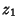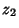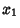## Box-Muller Transformation

A transformation which transforms from a 2-D continuous Uniform Distribution to a 2-D Gaussian Bivariate Distribution (or Complex Gaussian Distribution). Ifandare uniformly and independently distributed between 0 and 1, thenandas defined below have a Gaussian Distribution with Meanand Variance.(1)(2)

This can be verified by solving forand,(3)(4)

Taking the Jacobian yields(5)